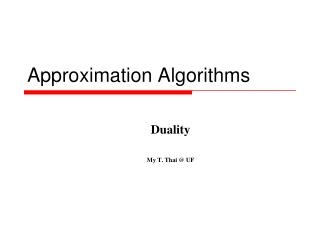DownloadDownload PresentationApproximation Algorithms

# Approximation Algorithms

Download Presentation## Approximation Algorithms

- - - - - - - - - - - - - - - - - - - - - - - - - - - E N D - - - - - - - - - - - - - - - - - - - - - - - - - - -
##### Presentation Transcript

1. Approximation Algorithms Duality My T. Thai @ UF

2. Duality • Given a primal problem: P: min cTx subject to Ax ≥ b, x ≥ 0 • The dual is: D: max bTy subject to ATy ≤ c, y ≥ 0 My T. Thai mythai@cise.ufl.edu

3. An Example My T. Thai mythai@cise.ufl.edu

4. Weak Duality Theorem • Weak duality Theorem: Let x and y be the feasible solutions for P and D respectively, then: • Proof: Follows immediately from the constraints My T. Thai mythai@cise.ufl.edu

5. Weak Duality Theorem • This theorem is very useful • Suppose there is a feasible solution y to D. Then any feasible solution of P has value lower bounded by bTy. This means that if P has a feasible solution, then it has an optimal solution • Reversing argument is also true • Therefore, if both P and D have feasible solutions, then both must have an optimal solution. My T. Thai mythai@cise.ufl.edu

6. Hidden Message ≥ • Strong Duality Theorem: If the primal P has an optimal solution x* then the dual D has an optimal solution y* such that: • cTx* = bTy* My T. Thai mythai@cise.ufl.edu

7. Complementary Slackness • Theorem: Let x and y be primal and dual feasible solutions respectively. Then x and y are both optimal iff two of the following conditions are satisfied: (ATy – c)j xj = 0 for all j = 1…n (Ax – b)i yi = 0 for all i = 1…m My T. Thai mythai@cise.ufl.edu

8. Proof of Complementary Slackness Proof: As in the proof of the weak duality theorem, we have: cTx ≥(ATy)Tx = yTAx ≥ yTb (1) From the strong duality theorem, we have: (2) (3) My T. Thai mythai@cise.ufl.edu

9. Proof (cont) Note that and We have: x and y optimal  (2) and (3) hold  both sums (4) and (5) are zero  all terms in both sums are zero (?)  Complementary slackness holds (4) (5) My T. Thai mythai@cise.ufl.edu

10. Why do we care? • It’s an easy way to check whether a pair of primal/dual feasible solutions are optimal • Given one optimal solution, complementary slackness makes it easy to find the optimal solution of the dual problem • May provide a simpler way to solve the primal My T. Thai mythai@cise.ufl.edu

11. Some examples • Solve this system: My T. Thai mythai@cise.ufl.edu

12. Min-Max Relations • What is a role of LP-duality • Max-flow and Min-Cut My T. Thai mythai@cise.ufl.edu

13. Max Flow in a Network • Definition: Given a directed graph G=(V,E) with two distinguished nodes, source s and sink t, a positive capacity function c: E → R+, find the maximum amount of flow that can be sent from s to t, subject to: • Capacity constraint: for each arc (i,j), the flow sent through (i,j), fij bounded by its capacity cij • Flow conservation:at each node i, other than s and t, the total flow into i should equal to the total flow out of i My T. Thai mythai@cise.ufl.edu

14. An Example 3 3 4 4 4 0 3 2 4 3 3 4 2 1 2 1 t s 1 3 1 1 3 2 0 2 3 0 2 1 2 0 5 0 My T. Thai mythai@cise.ufl.edu

15. Formulate Max Flow as an LP • Capacity constraints: 0 ≤ fij ≤ cij for all (i,j) • Conservation constraints: • We have the following: My T. Thai mythai@cise.ufl.edu

16. LP Formulation (cont) 3 3 4 4 4 0 3 2 4 3 4 3 2 1 3 2 1 t 1 s 1 1 2 3 2 0 3 1 0 2 2 0 5 0 ∞ My T. Thai mythai@cise.ufl.edu

17. LP Formulation (cont) My T. Thai mythai@cise.ufl.edu

18. Min Cut • Capacity of any s-t cut is an upper bound on any feasible flow • If the capacity of an s-t cut is equal to the value of a maximum flow, then that cut is a minimum cut My T. Thai mythai@cise.ufl.edu

19. Max Flow and Min Cut My T. Thai mythai@cise.ufl.edu

20. Solutions of IP Consider: Let (d*,p*) be the optimal solution to this IP. Then: • ps* = 1 and pt* = 0. So define X = {pi | pi = 1} and X = {pi | pi = 0}. Then we can find the s-t cut • dij* =1. So for i in X and j in X, define dij = 1, otherwise dij = 0. • Then the object function is equal to the minimum s-t cut My T. Thai mythai@cise.ufl.edu

21. LP-relaxation • Relax the integrality constraints of the previous IP, we will obtain the previous dual. My T. Thai mythai@cise.ufl.edu

22. Design Techniques • Many combinatorial optimization problems can be stated as IP • Using LP-relaxation techniques, we obtain LP • The feasible solutions of the LP-relaxation is a factional solution to the original. However, we are interested in finding a near-optimal integral solution: • Rounding Techniques • Primal-dual Schema My T. Thai mythai@cise.ufl.edu

23. Rounding Techniques • Solve the LP and convert the obtained fractional solution to an integral solution: • Deterministic • Probabilistic (randomized rounding) My T. Thai mythai@cise.ufl.edu

24. Primal-Dual Schema • An integral solution of LP-relaxation and a feasible solution to the dual program are constructed iteratively • Any feasible solution of the dual also provides the lower bound of OPT • Comparing the two solutions will establish the approximation guarantee My T. Thai mythai@cise.ufl.edu